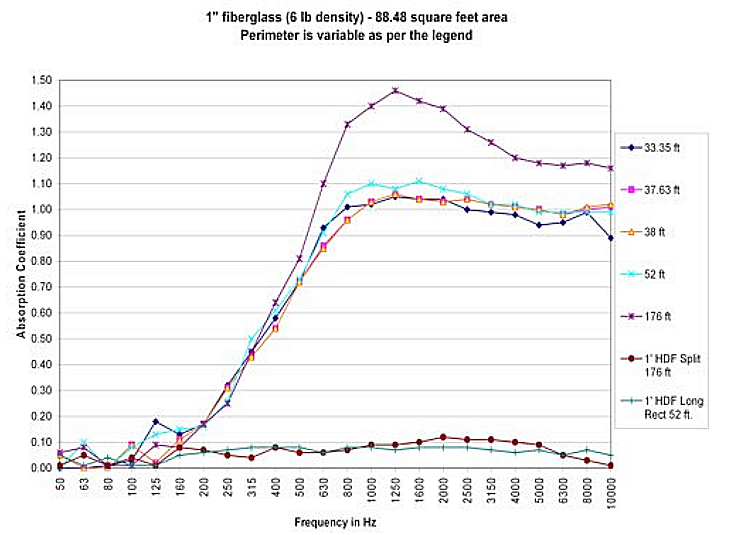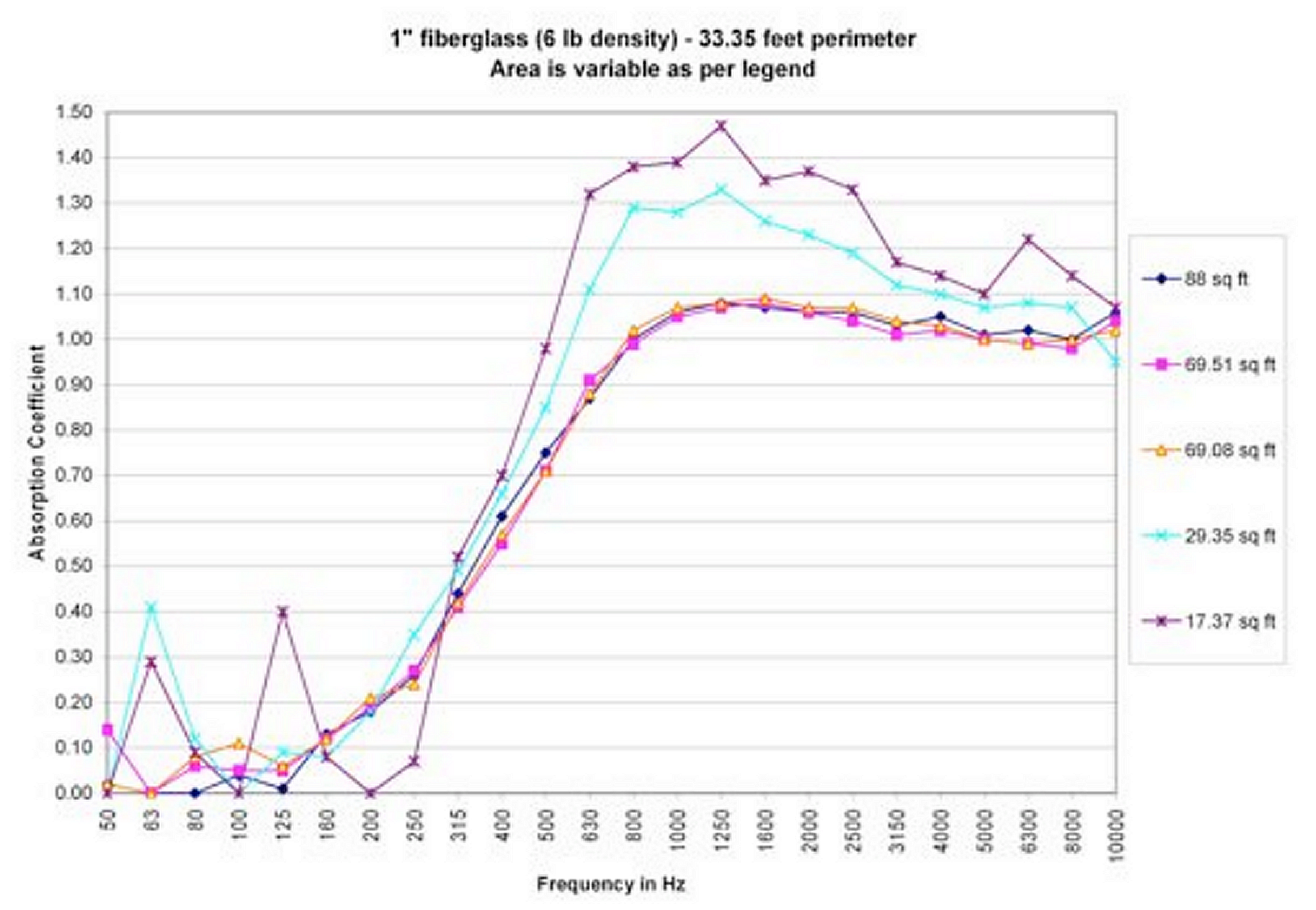# RT and the Area of Acoustical Absorption

By Richard Honeycutt

## This article explains collation between reverberation time (RT) and the area of acoustical absorption.

The Sabine equation for calculating reverberation time (RT) is:

RT = 0.049V/A

• where V is the volume of the room in cubic feet,
• and A is the total acoustical absorption in the room, in equivalent square feet of perfect absorber.

We quantify A in Imperial or metric sabins, ft2, or m2.

The absorption in sabins is the sum of the products of the areas of different materials in the room and their individual absorption coefficients (sabins/square foot), for which we now use the symbol α. Thus Sabine said that an α of 0.5 represents a material that absorbs 50% of the sound striking it. Sabine believed that the α depended entirely upon the nature of the material: surface porosity, internal friction, thickness, etc. To the extent that these factors varied with frequency, so would the α.

Although Sabine’s theory was the first step in establishing the science of architectural acoustics, it is not entirely precise in its theory, or universal in its applicability. First, it is based upon a statistical approach to acoustics that assumes that the room is a diffuse space (no acoustical focusing) with the same RT at every point, and that the absorption is evenly distributed among all surfaces (walls, floor, and ceiling). No doubt Sabine knew, as we do today, that these assumptions are not met in all rooms.

What Sabine may not have considered is the errors introduced by treating sound—longitudinal waves in air—as geometric rays. To this day, we often fail to recognize the effects of this oversimplification, even though we try to improve our predictions by using scattering.

The first shoe to drop was the determination that, when measured under standard conditions, some materials seem to have α’s that exceed 1.0: they seem to absorb more than 100% of the incident sound. When measured results do not make sense according to known physical principles, we look for unaccounted-for variables. In this case, the stock answer to “how can this be?” has been that it is accounted for by “edge effect”. Answers to “so what is ‘edge effect’ and how do we account for it?” have been less forthcoming. The answer seems to be threefold:

(1) The statistical assumptions upon which Sabine theory is based are violated in practice. When a sound field encounters the edge of a piece of absorptive material, the acoustical impedance near the material changes, causing wave refraction, with the result that the material can appear as much as ¼ wavelength larger in each dimension than it actually is. This makes near-grazing waves strike the absorber and be partially absorbed. (http://www.sengpielaudio.co/AbsorptionsgradGroesserEins.pdf)

(2) Edge diffraction causes sound crossing an edge of an absorber to change direction. This also causes an effect similar to that of a larger area of absorption. The way this works can be understood with the aid of Huygens’ principle: every point illuminated by a wave can be considered as a spherical-wave source. Thus when an edge is irradiated by sound, the sound beyond that edge can be calculated as a superposition of all these spherical sources. This means that we can imagine a line of spherical radiators at the edge of the absorptive material in Figure 1 and thus calculate the strength of the diffracted sound in any location. Since the radiation is spherical, sound is transmitted in all directions, including along the vertical and horizontal surfaces of the absorber’s edge. In the case of diffraction, the amount by which the absorber’s effective area increases is proportional to the ratio of the perimeter of the edges to the area of the absorber.

(3) Beranek pointed out that if α represents the percentage of incident sound absorbed by a surface, then a room whose surfaces all had α=1 would have an RT of zero seconds, since there would be no energy in the reverberant field. In fact, to obtain an RT of zero using the Sabine equation, the α would have to equal infinity. Thus α values >1 are real; it’s just that we have misunderstood what α actually is.

S-I Thomasson investigated the effect of area upon sound absorption. (“Theory and Experiments on the sound absorption as a function of area.” Report TRITA-TAK 8201, Royal Institute of Technology, Sweden, 1982.) He developed models for including the effects of absorber size and the angle of sound incidence.

At InterNoise 2009, Ron Sauro of NWAA Labs presented the results of measurements of the RT in a test lab with absorptive material of a given area, but measured first in a single piece, then cut into pieces so that the area could be held constant while the perimeter varied. Figures 2 and 3 show the results of the measurements.Figure 2: Constant area, variable perimeter (http://nwaalabs.ipower.com/Files/NWAA%20Labs/IN09_092.pdf)Figure 3: Constant perimeter, variable area (http://nwaalabs.ipower.com/Files/NWAA%20Labs/IN09_092.pdf)

Notice that α varies with both area and perimeter, and α varies substantially with frequency, particularly above 250 Hz and for very absorptive materials.

Sauro’s paper includes suggested methods for incorporating the perimeter into a modeling calculation.

So what can we say right now? We can say that in Sabine theory, the wavelength of incident sound does not affect acoustical absorptivity. However, in working with actual pieces of very absorptive material having specific areas and perimeters, we must consider both the area and the perimeter in order to accurately predict the net acoustical absorption. This welcome quantification of the “mysterious” edge effect explains the oft-given advice that absorptive material used for reverberation control behaves more efficiently if used in scattered blocks rather than single large pieces. We can say that longer-wavelength sound does not require larger areas of absorptive material, since the edge effect is mainly important at higher frequencies. This last statement is easier to wrap your head around if you remember that sound waves are longitudinal, not transverse, so a longer wavelength does not mean that a larger area of absorption is impacted when sound strikes it. And we can say that a given area of absorptive material will absorb more total sound if applied in smaller pieces, rather than a single large piece. rh